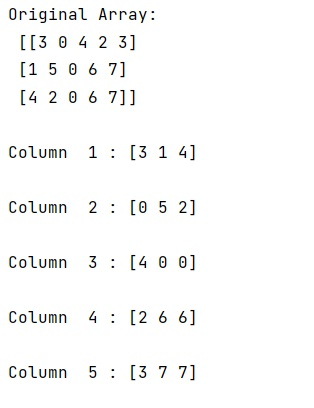# NumPy: How to iterate over columns of array?

Given a NumPy array, we have to iterate over columns of array.
Submitted by Pranit Sharma, on December 24, 2022

NumPy is an abbreviated form of Numerical Python. It is used for different types of scientific operations in python. Numpy is a vast library in python which is used for almost every kind of scientific or mathematical operation. It is itself an array which is a collection of various methods and functions for processing the arrays.

## Iterating over columns of NumPy array

Suppose we are given a M X N array and we need to pass each of its columns into a function to perform some operation on the entire column, so for this purpose, we need to learn how to iterate over the columns of an array.

For this purpose, we just need to use the transpose of the array which is an iterable object hence we will loop over the transpose of an array, and at each iteration, we will access each column of the array.

Transpose is a concept of the matrix which we use to cross the rows and columns of the 2-dimensional array or a matrix or a DataFrame.

For finding a transpose of an array, we just need to use the array.T method and to iterate over each we will use the following code snippet:

```for col in arr.T
```

Let us understand with the help of an example,

## Python code to iterate over columns of array

```# Import numpy

import numpy as np

# Creating a numpy array
arr = np.array([[3, 0, 4, 2, 3],[ 1, 5, 0, 6, 7],[ 4, 2, 0, 6, 7]])

# Display original array
print("Original Array:\n",arr,"\n")

# Accessing columns of arr
i=1
for col in arr.T:
print("Column ",i,":", col,"\n")
i+=1
```

Output: